﻿ Undetected Error Probability for Quantum Codes

### Undetected Error Probability for Quantum Codes

Manish Gupta, R.K. Narula, Divya TanejaOPEN ACCESSPEER-REVIEWED

## Undetected Error Probability for Quantum Codes

Manish Gupta1, R.K. Narula2, Divya Taneja3, 4,1Baba Farid College of Engineering & Technology Bathinda, Punjab, India

2PIT, Mansa, Punjab, India

3Yadavindra College of Engineering, Punjabi University Guru Kashi Campus, Talwandi Sabo, Punjab, India

4Research Scholar Punjab Technical University, Jalandhar, Punjab, India

### Abstract

From last fourteen years the work on undetected error probability for quantum codes has been silent. The undetected error probability has been discussed by Ashikhmin  in which it was proved that the average probability of undetected error for a given code is essentially given by a function of its weight enumerators. In this paper, new upper bounds on undetected error probability for quantum codes used for error detection on depolarization channel are given. It has also been established that the probability of undetected errors for quantum codes over depolarization channel do satisfy the upper bound analogous to classical codes.

• Gupta, Manish, R.K. Narula, and Divya Taneja. "Undetected Error Probability for Quantum Codes." American Journal of Applied Mathematics and Statistics 3.2 (2015): 76-79.
• Gupta, M. , Narula, R. , & Taneja, D. (2015). Undetected Error Probability for Quantum Codes. American Journal of Applied Mathematics and Statistics, 3(2), 76-79.
• Gupta, Manish, R.K. Narula, and Divya Taneja. "Undetected Error Probability for Quantum Codes." American Journal of Applied Mathematics and Statistics 3, no. 2 (2015): 76-79.

 Import into BibTeX Import into EndNote Import into RefMan Import into RefWorks

### 1. Introduction

With the discovery of Shor’s algorithm, Quantum computing has become an active interdisciplinary field of research. Quantum computers are able to solve hard computational problems more efficiently than present classical computers. But reliability of the quantum computers is questionable since the quantum states are subjected to decoherence. Quantum error correcting codes are the means of protecting quantum information against external sources such as noise and decoherence. Many explicit constructions of quantum error-correcting codes have been proposed so far. Most of the codes known so far are additive or stabilizer codes which are constructed from classical binary code that are self-orthogonal with respect to a certain symplectic inner product. An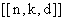code is an additive quantum code of minimum-distance d of length n encoding k quantum bits and an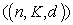code refers to a general code encoding K states in n qubits with minimum distance d. A code is called nonadditive if it is not equivalent to any additive code.

The construction of additive quantum codes using additive classical codes C over GF(4) is given in . An important class of quantum codes called Stabilizer codes is defined in  and  which are analogous to the quantum additive codes. Among the additive codes the minimum distance two codes are those which correct any single qubit erasure. These distance two codes have been extensively studied and several constructions of both additive and nonadditive distance 2 codes are available in [1, 2, 5, 7, 8, 9, 11]. In our earlier work , we have also studied these distance 2 codes and now are in a position to find their undetected error probability.

In classical coding theory decoding is done by observing the received vector. If the received vector is not contained in the code space then an error is detected. An error remains undetected if the sent vector and the error vector sum up to a code word in the code space itself. The probability of undetected error for a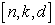code is given by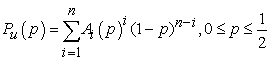where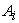is the number of code words of weight i in code. It was shown in  that the undetected error probability, when used solely for error detection on binary symmetric channel with crossover probability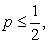is upper bounded by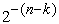. In quantum case, the error will not be detected if the measured transmission results in the code itself and is not orthogonal or collinear to transmitted state vector. The probability of undetected error in this case, as shown by  can be computed via the weight enumerators of quantum codes. For a stabilizer code this probability is given by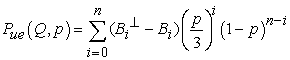where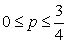and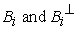are the weight distributions of the quantum codes as defined in .

The optimal distance 2 codes along with their stabilizer structures and their explicit basis were found in our earlier work . In this paper, the probability of undetected error for both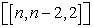and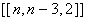code have been found. This probability function is further proved to be monotonic increasing having an upper boundwhich is same as classical codes as given in .

### 2. Probability of Undetected Error

In  we have shown that the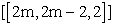code is constructed from a classical additive self dual code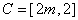whose generator matrix is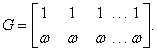The direct sum of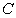with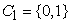is used to construct the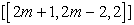code whose generator matrix is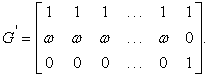In this paper, the probability of undetected error for both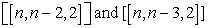code have been found. This probability function is further proved to be monotonic increasing having an upper boundwhich is same as classical codes.

2.1. Undetected Error Probability for Even Length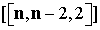Quantum Code

The weight enumerators of the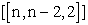quantum code are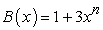and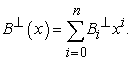Also by MacWilliams Identity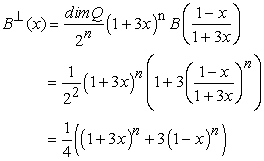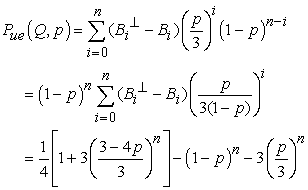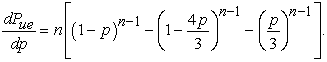We shall prove by induction that for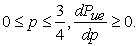Now for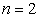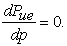Let us assume for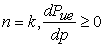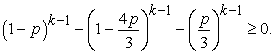Now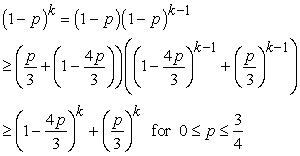Thus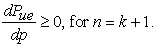Thus by induction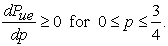and hence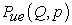s an increasing function in this interval.

Now for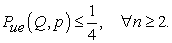Thusis upper bounded by.

2.2. Undetected Error Probability of Odd Length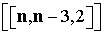Quantum Code

The odd length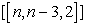quantum codes are obtained by taking the direct sum of the classical even length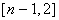code C overwith.

Now from , the weight enumerator of the resulting classical code will be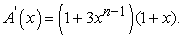Hence, the weight enumerator of the quantum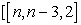code will be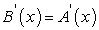and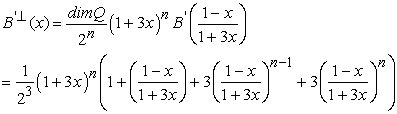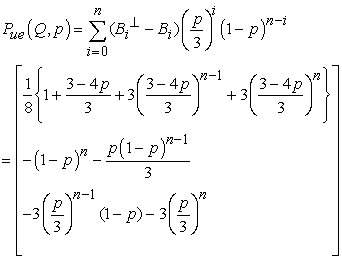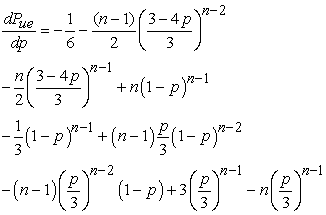We shall prove that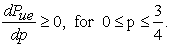Now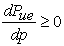if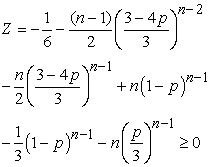When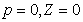.

Let us assume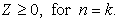That is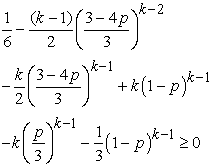We shall prove that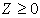for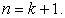Now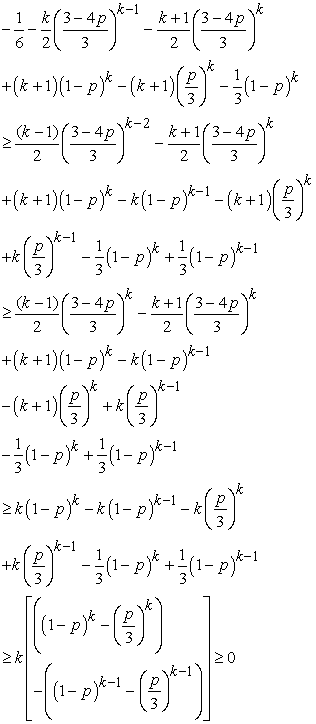Thus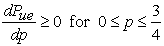and henceis an increasing function in this interval.

Now for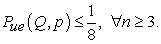Thus theis upper bounded by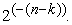Thus we have proved that quantumandcodes obeys the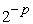bound, where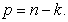This result is similar to the general rule for the classical linear block codes as given in .

2.2.1. Remark

We have also verified that this bound is satisfied by the quantum Hamming codes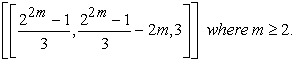The probability of undetected error of these codes is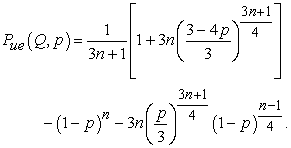This is a monotonic increasing function of p giving an upper bound. Many more quantum codes satisfy this bound.

In classical codes there are certain codes , in which this bound is not satisfied so such violation in quantum additive codes would be the topic of further investigation

### 3. Conclusion

The probability of undetected error for optimal distance 2 codes was found to be increasing functions and satisfies the upper boundwhich is same as the classical bound.

### Acknowledgement

This research work is supported by National Board for Higher Mathematics (NBHM), Mumbai with Ref. No. 2/48(1)/2012/NBHM/R&D11/10924.

One of the author, Divya Taneja also acknowledges Punjab Technical University, Jalandhar, Punjab for providing the research facilities.

### References

  Calderbank, A., Rains, E. M., Shor, P. W. and Sloane, N. J. A., “Quantum error correction via codes over GF(4),” IEEE Trans. Inf. Theory, vol. 44, pp. 1369-1387,1998.In article CrossRef  Cross, A., Smith, G., Smolin, J.A. and Zeng, B., “Codeword Stabilized Quantum Codes.” IEEE Trans. Inf. Theory,vol.55, pp. 433-438, 2009.In article CrossRef  Ashikhmin, A. E., Barg, A. M., Knill, E.and Litsyn, S. N., “Quantum Error Detection I: Statement of the Problem”, IEEE Trans. Inf. Theory, vol. 46, no. 3, pp. 778-788, 2000.In article CrossRef  Gottesman, D., “Stabilizer Codes and Quantum Error Correction,” Caltech Ph.D. dissertation, California Institute of Technology, Psadena, CA, 1997.In article  Rains, E. M., “Quantum codes of minimum distance two,” IEEE Trans. Inf. Theory, vol. 45, no. 1, pp. 266-271, 1999.In article CrossRef  Rains, E. M., “Quantum shadow enumerators”, IEEE Trans. Inf. Theory, vol. 45, no. 7, pp. 2361- 2366, 1999.In article CrossRef  Rains, E. M., Hardin, R. H., Shor, P. W. and Sloane, N. J. A.,“A nonadditive quantum code,” Phys. Rev. Lett., vol. 79, pp. 953-954,1997.In article CrossRef  Smolin, J. A., Smith, G., and Wehner, S., “A simple family of nonadditive quantum codes” arXiv: quant-ph/0701065v2, 2007.In article  Feng, K. and Xing, C. P., “A new construction on quantum error-correcting codes,” Trans. Amer. Math. Soc., vol. 360, pp. 2007-2019, 2008.In article CrossRef  Shor, P. W. and Laflamme, R., “Quantum analog of the MacWilliams identities in classical coding theory,” Phys. Rev. Lett., vol. 78, pp. 1600-1602, 1997.In article CrossRef  Aggarwal, V. and Calderbank, R., “Boolean Functions, Projection Operators and Quantum Error Correcting Codes.”IEEE Trans. Inf. Theory, vol.54, pp. 1700-1707, 2008.In article CrossRef  Cary Huffman, W. and Vera Pless, “Fundamentals of Error-Correcting Codes.” Cambridge University Press, 2003.In article  Leung-Yan-Cheong, S. K. and Hellman, M.E. “Concerning a bound on undetected error probability,” IEEE Trans. Inf. Theory, vol. IT- 22. pp. 235-231, 1976.In article  Gupta, M., Narula, R. K and Taneja, D., “On the Construction of Odd Length Quantum Codes,”British Journal of Mathematics & Computer Science 6(5), pp. 444-450, 2015.In article CrossRef